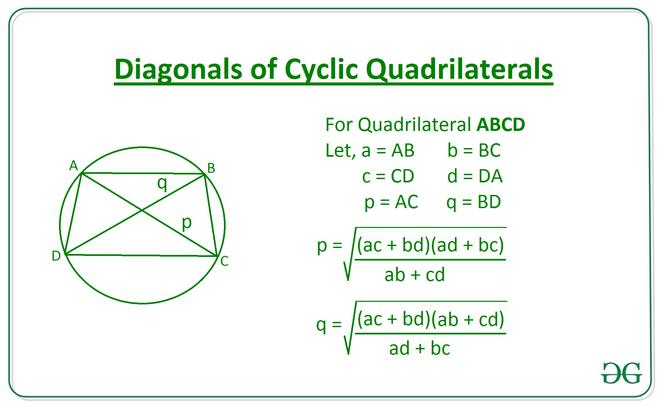# Length of Diagonals of a Cyclic Quadrilateral using the length of Sides.

Given integers A, B, C, and D, denoting the length of sides of a Cyclic Quadrilateral, the task is to find the length of diagonals of a cyclic quadrilateral.

Examples:

Input: A = 10, B = 15, C = 20, D = 25
Output: 22.06 26.07
Input: A = 10, B = 30, C =50, D = 20
Output: 37.93 29.0

Approach: The length of diagonals can be calculated using the following equations:[Tex]Diagonal (q)=\sqrt{\frac{(ac+bd)(ab+cd)}{ad+bc}}         [/Tex]Below is the implementation of the above approach:

## C++

 // C++ Program to implement // the above approach #include  using namespace std;   // Function to calcualte the length of // diagonals of a cyclic quadrilateral vector<float> Diagonals(int a, int b,                          int c, int d) {     vector<float> ans;     ans.push_back(sqrt(((a * c) + (b * d)) *                         ((a * d) + (b * c)) /                         ((a * b) + (c * d))));     ans.push_back(sqrt(((a * c) + (b * d)) *                         ((a * b) + (c * d)) /                         ((a * d) + (b * c))));     return ans; }   // Driver Code int main() {     int A = 10;     int B = 15;     int C = 20;     int D = 25;       // Function Call     vector<float> ans = Diagonals(A, B, C, D);       // Print the final answer     printf("%.2f %.2f",             (ans) + .01,              ans + .01); }   // This code is contributed by Amit Katiyar

## Java

 // Java Program to implement // the above approach import java.util.*; class GFG{   // Function to calcualte the length of // diagonals of a cyclic quadrilateral static Vector Diagonals(int a, int b,                                 int c, int d) {     Vector ans = new Vector();     ans.add((float) Math.sqrt(((a * c) + (b * d)) *                                ((a * d) + (b * c)) /                                ((a * b) + (c * d))));     ans.add((float) Math.sqrt(((a * c) + (b * d)) *                                ((a * b) + (c * d)) /                                ((a * d) + (b * c))));     return ans; }   // Driver Code public static void main(String[] args) {     int A = 10;     int B = 15;     int C = 20;     int D = 25;       // Function Call     Vector ans = Diagonals(A, B,                                    C, D);       // Print the final answer     System.out.printf("%.2f %.2f",                        (ans.get(0)) + .01,                         ans.get(1) + .01); } }   // This code is contributed by 29AjayKumar

## Python3

 # Python3 program to implement # the above approach   import math   # Function to calcualte the length of # diagonals of a cyclic quadrilateral def Diagonals(a, b, c, d):       p = math.sqrt(((a * c)+(b * d))*((a * d)+(b * c))                   / ((a * b)+(c * d)))     q = math.sqrt(((a * c)+(b * d))*((a * b)+(c * d))                   / ((a * d)+(b * c)))       return [p, q]     # Driver Code A = 10 B = 15 C = 20 D = 25   # Function Call ans = Diagonals(A, B, C, D)   # Print the final answer print(round(ans, 2), round(ans, 2))

## C#

 // C# Program to implement // the above approach using System; using System.Collections.Generic; class GFG{   // Function to calcualte the length of // diagonals of a cyclic quadrilateral static List<float> Diagonals(int a, int b,                               int c, int d) {   List<float> ans = new List<float>();   ans.Add((float) Math.Sqrt(((a * c) + (b * d)) *                              ((a * d) + (b * c)) /                              ((a * b) + (c * d))));   ans.Add((float) Math.Sqrt(((a * c) + (b * d)) *                              ((a * b) + (c * d)) /                              ((a * d) + (b * c))));   return ans; }   // Driver Code public static void Main(String[] args) {   int A = 10;   int B = 15;   int C = 20;   int D = 25;     // Function Call   List<float> ans = Diagonals(A, B,                                C, D);     // Print the readonly answer   Console.Write("{0:F2} {1:F2}",                   (ans) + .01,                    ans + .01); } }    // This code is contributed by 29AjayKumar

Output:

22.06 26.07



Time Complexity: O(1)
Auxiliary Space: O(1)

Attention reader! Don’t stop learning now. Get hold of all the important DSA concepts with the DSA Self Paced Course at a student-friendly price and become industry ready.

My Personal Notes arrow_drop_upCheck out this Author's contributed articles.

If you like GeeksforGeeks and would like to contribute, you can also write an article using contribute.geeksforgeeks.org or mail your article to contribute@geeksforgeeks.org. See your article appearing on the GeeksforGeeks main page and help other Geeks.

Please Improve this article if you find anything incorrect by clicking on the "Improve Article" button below.